# Class 9 RD Sharma Solutions – Chapter 15 Areas of Parallelograms and Triangles- Exercise 15.3 | Set 3

### Question 21. In figure, PSDA is a parallelogram in which PQ = QR = RS and AP || BQ || CR. Prove that ar (ΔPQE) = ar(ΔCFD).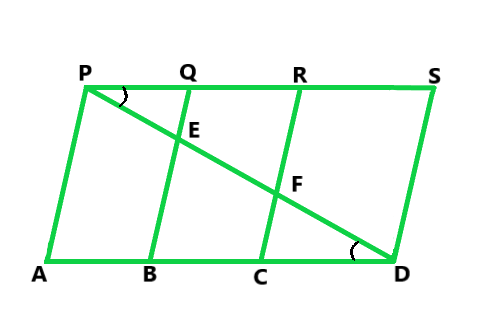Solution:

According to the question

PSDA is a parallelogram

So, AP || BQ || CR || DS and AD || PS

PQ = CD       …..(i)

Prove that (ΔPQE) = ar(ΔCFD)

Proof:

In ΔBED,

C is the midpoint of BD and CF || BE

So, F is the midpoint of ED

Hence, EF = PE

Similarly, EF = PE

Therefore, PE = FD             …..(ii)

In ΔPQE and CFD,

PE = FD

∠ EPQ = ∠FDC                            (Alternate angles)

PQ = CD

So, by SAS congruence, we have

ΔPQE ≅ ΔDCF

Hence, ar(ΔPQE) = ar(ΔDCF)

### Question 22. In figure, ABCD is a trapezium in which AB || DC and DC = 40 cm and AB = 60 cm. If X and Y are, respectively, the mid-points of AD and BC, prove that:

(i) XY = 50 cm

(ii) DCYX is a trapezium

(iii) ar(trap. DCYX) = (9/11) ar(XYBA).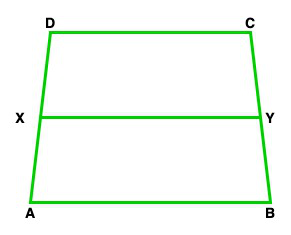Solution:

(i) Join DY and produce it to meet AB produced at P.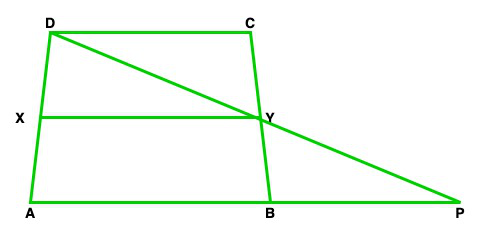In ΔBYP and CYD

∠BYP = ∠ CYD          (Vertically opposite angles)

∠DCY = ∠ PBY           (As, DC || AP)

BY = CY

So, by ASA congruence,

ΔBYP ≅ ΔCYD

By C.P.C.T

DY = YP and DC = BP

As we know that, Y is the midpoint of DP

also, X is the midpoint of AD

So, XY || AP and XY || (1/2) AP

XY = (1/2) (AB + BP)

XY = (1/2) (AB + DC)

XY = (1/2) (60 + 40) = 50 cm

(ii) Given that,

XY || AP

XY || AB and AB || DC

XY || DC

Hence, DCYX is a trapezium

(iii) As we know that X and Y are the mid-points of Ad and BC.

So, trapezium DCYX and ABYX are of the same height

Let us assume, the height of the trapezium is h cm

Now,

ar(trap. DCXY) = (1/2)(DC + XY) × h

ar(trap. DCXY) = (1/2) (50 + 40) × h cm2 = 45 h cm2

ar(trap. ABYX) = (1/2)(AB + XY) × h

ar(trap. ABYX) = (1/2)(60 + 50) × h cm2 = 55h cm2

ar(trap. DCYX) ar(trap. ABYX) = 45h/55h = 9/11

Hence, ar(trap. DCYX) = 9/11 ar(trap. ABYX)

### Question 23. In figure, ABC and BDE are two equilateral triangles such that D is the midpoint of BC. AE intersects BC in F. Prove that:

(i) ar(ΔBDE) = (1/4) ar(ΔABC)

(ii) ar(ΔBDE) = (1/2) ar(ΔBAE)

(iii) ar(ΔBFE) = ar(ΔAFD)

(iv) ar(ΔABC) = 2 ar(ΔBEC)

(v) ar(ΔFED) = 1/8 ar(ΔAFC)

(vi) ar(ΔBFE) = 2 ar(ΔEFD)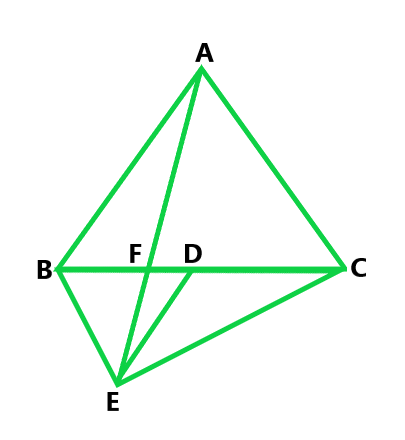Solution:

According to the question

ABC and BDE are two equilateral triangles.

Let us considered AB = BC = CA = x. Then, BD = x/2 = DE = BE

(i) Given that,

ar(ΔABC) = √3/4 x2 ….(i)

ar(ΔBDE) = √3/4 (x/2)2 = 1/4 x √3/4 x2

Now put the value of √3/4 x2 from eq(i), we get

Hence, ar(ΔBDE) = 1/4 ar(ΔABC)

Hence proved

(ii) Given that,

ΔABC and BED are equilateral triangles

So, ∠ ACB = ∠DBE = 60°

BE || AC                                    (Alternative angles are equal)

As we know that ΔBAF and ΔBEC are on the same base BE

and between same parallels BF and AC.

So, ar(ΔBAE) = ar(ΔBEC)

ED is a median of ΔEBC

So, ar(ΔBAE) = 2ar(ΔBDE)

Hence, ar(ΔBDE) = (1/2) ar(ΔBAE)

(iii) Given that,

ΔABC and BDE are equilateral triangles

So, ∠ABC = 60° and ∠BDE = 60°

∠ABC = ∠BDE

AB || DE                   (Alternate angles are equal)

As we know that ΔBED and ΔAED are on the same base ED and

between same parallels AB and DE.

So, ar(ΔBED) = ar(ΔAED)

ar(ΔBED) − ar(ΔEFD) = ar(ΔAED) − ar(ΔEFD)

Hence, ar(ΔBEF) = ar(ΔAFD)

(iv) Given that,

ED is the median of tΔBEC

So, ar(ΔBEC) = 2ar(ΔBDE)

ar(ΔBEC) = 2 × (1/2) ar(ΔABC)    (Proved above)

ar(ΔBEC) = (1/2) ar(ΔABC)

Hence, ar(ΔABC) = 2ar(ΔBEC)

(v) ar(ΔAFC) = ar(ΔAFD) + ar(ΔADC)

ar(ΔBFE) + (1/2) ar(ΔABC)

ar(ΔBFE) + (1/2) × 4ar(ΔBDE)

ar(ΔBFE) = 2ar(ΔFED)  …..(iii)

ar(ΔBDE) = ar(ΔBFE) + ar(ΔFED)

2ar(ΔFED) + ar(ΔFED)

3ar(ΔFED)         ….(iv)

From eq (ii), (iii) and (iv), we get

ar(ΔAFC) = 2ar(ΔFED) + 2 × 3 ar(ΔFED) = 8 ar(ΔFED)

Hence, ar(ΔFED) = (1/8) ar(ΔAFC)

(vi) Let’s assume that h be the height of vertex E, corresponding to the side BD in ΔBDE and

H be the height of vertex A, corresponding to the side BC in ΔABC

As we proved above

ar(ΔBDE) = (1/4) ar(ΔABC)

(1/2) × BD × h = (1/4) (1/2 × BC × h)

BD × h = (1/4)(2BD × H)

h = (1/2) H    ….(i)

From part (iii), we get

ar(ΔBFE) = ar(ΔAFD)

ar(ΔBFE) = (1/2) × FD × H

ar(ΔBFE) = (1/2) × FD × 2h

ar(ΔBFE) = 2((1/2) × FD × h)

Hence, ar(ΔBFE) = 2ar(ΔEFD)

### Question 24. D is the midpoint of side BC of ΔABC and E is the midpoint of BD. If O is the midpoint of AE, Prove that ar(ΔBOE) = (1/8) ar(ΔABC).

Solution:

According to the question

D is the midpoint of sides BC of Δ ABC,

E is the midpoint of BD and O is the midpoint of AE,

So, AD and AE are the medians of ΔABC and ΔABD

Hence, ar(ΔABD) = (1/2) ar(ΔABC)   …..(i)

and ar(ΔABE) = (1/2) ar(ΔABD)   …….(ii)

Also, OB is the median of triangle ABE

So, ar(ΔBOE) = (1/2) ar(ΔABE)

From eq (i), (ii) and (iii), we conclude that

ar(ΔBOE) = (1/8) ar(ΔABC)

Hence proved

### Question 25. In figure, X and Y are the mid points of AC and AB respectively, QP || BC and CYQ and BXP are straight lines. Prove that ar(ΔABP) = ar(ΔACQ).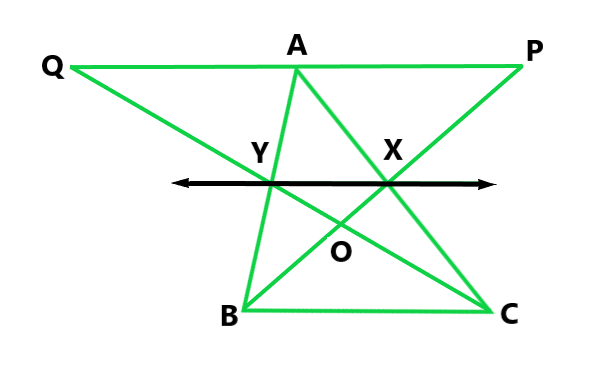Solution:

According to the question

X and Y are the mid-points of AC and AB

So, XY || BC

Prove: ar(ΔABP) = ar(ΔACQ)

Proof:

From the figure, we conclude that ΔBYC and BXC are on the same base BC and

between the same parallels XY and BC

So, ar(ΔBYC) = ar(ΔBXC)

ar(ΔBYC) − ar(ΔBOC) = ar(ΔBXC) − ar(ΔBOC)

ar(ΔBOY) = ar(ΔCOX)

ar(ΔBOY) + ar(ΔXOY) = ar(ΔCOX) + ar(ΔXOY)

ar(ΔBXY) = ar(ΔCXY)             …..(i)

From the figure we conclude that the quadrilaterals XYAP and XYAQ are on

the same base XY and between same parallels XY and PQ.

Now, add eq(i) and (ii), we get

ar(ΔABP) = ar(ΔACQ)

Hence proved

### Question 26. In figure, ABCD and AEFD are two parallelograms. Prove that

(i) PE = FQ

(ii) ar(ΔAPE) : ar(ΔPFA) = ar(ΔQFD) : ar(ΔPFD)

(iii) ar(ΔPEA) = ar(ΔQFD)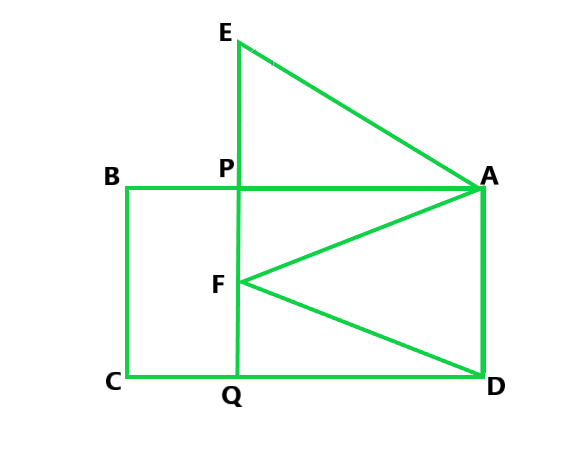Solution:

According to the question

ABCD and AEFD are two parallelograms

(i) Prove that PE = FQ

Proof:

In ΔEPA and FQD

∠PEA = ∠QFD         (Corresponding angles)

∠EPA=∠FQD           (Corresponding angles)

PA = QD                   (Opposite sides of parallelogram)

So, by AAS congruence

ΔEPA ≅ ΔFQD

Hence, by C.P.C.T

EP = FQ

Hence proved

(ii) Prove that ar(ΔAPE) : ar(ΔPFA) = ar(ΔQFD) : ar(ΔPFD)

From the figure we conclude that ΔPEA and ΔQFD stand on equal bases

PE and FQ lies between the same parallels EQ and AD

So, ar(ΔPEA) = ar(ΔQFD) ……(i)

From the figure we conclude that ΔPEA and ΔPFD stand on the same

base PF and between same parallels PF and AD

Therefore, ar(ΔPFA) = ar(ΔPFD)      ……(ii)

Now divide eq(i) by eq(ii), we get

Hence, ar(ΔPEA) : ar(ΔPFA) = ar(ΔQFD) : ar(ΔPFD)

Hence proved

(iii) Prove that ar(ΔPEA) = ar(ΔQFD)

Proof:

As we proved above that

ΔEPA ≅ ΔFQD

Hence, ar(ΔPEA) = ar(ΔQFD)

Hence proved

### Question 27. In figure, ABCD is a parallelogram. O is any point on AC. PQ || AB and LM || AD. Prove that: ar(||gm DLOP) = ar(||gm BMOQ).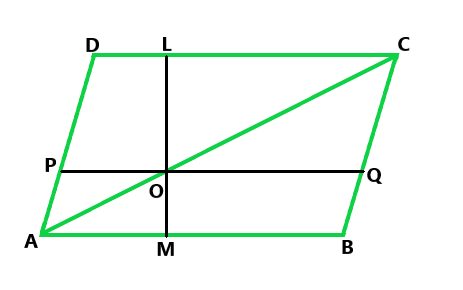Solution:

According to the question

ABCD is a parallelogram and PQ || AB and LM || AD

Prove that ar(||gm DLOP) = ar(||gm BMOQ)

Proof:

Here, the diagonal AC of a parallelogram divides it into two triangles of equal area

ar(ΔAPO) + ar(||gm DLOP) + ar(ΔOLC)

ar(ΔAOM) + ar(||gm BMOQ) + ar(ΔOQC)           ……(i)

From the figure, AO and OC are diagonals of parallelograms AMOP and OQCL

So, ar(ΔAPO) = ar(ΔAMO)             ……(ii)

And ar(ΔOLC) = ar(ΔOQC)            ……(iii)

Now subtracting eq(ii) and (iii) from (i), we get

ar(||gm DLOP) = ar(||gm BMOQ)

Hence proved

### Question 28. In a triangle ABC, if L and M are points on AB and AC respectively such that LM || BC. Prove that:

(i) ar(ΔLCM) = ar(ΔLBM)

(ii) ar(ΔLBC) = ar(ΔMBC)

(iii) ar(ΔABM) = ar(ΔACL)

(iv) ar(ΔLOB) = ar(ΔMOC)

Solution:

According to the question

ABC is a triangle and L and M are points on AB and AC respectively such that LM || BC

(i) Here, Δ LMB and LMC are on the same base LM and between the same parallels LM and BC.

Hence, ar(ΔLMB) = ar(ΔLMC)

(ii) Here, ΔLBC and MBC are on the same base BC and between same parallels LM and BC.

Hence, ar(ΔLBC) = ar(ΔMBC)

(iii) Here, we have

ar(ΔLMB) = ar(ΔLMC)            (Proved above)

ar(ΔALM) + ar(ΔLMB) = ar(ΔALM) + ar(ΔLMC)

Hence, ar(ΔABM) = ar(ΔACL)

(iv) Here, we have

ar(ΔLBC) = ar(ΔMBC)              (Proved above)

ar(ΔLBC) − ar(ΔBOC) = ar(ΔMBC) − ar(ΔBOC)

Hence, ar(ΔLOB) = ar(ΔMOC).

### Question 29. In figure, D and E are two points on BC such that BD = DE = EC. Show that ar(ΔABD) = ar(ΔADE) = ar(ΔAEC).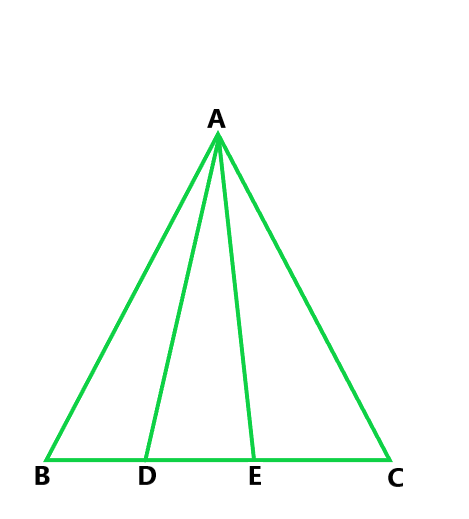Solution:

In the triangle ABC, draw a line || through A parallel to BC.

BD = DE = EC  (Given)

Now from the figure, triangles ABD and AEC are on the same base AC and

between the same parallels l and BC. Hence, the area of triangles ABD and AEC is equal.

Hence, ar(ΔABD) = ar(ΔADE) = ar(ΔAEC).

### Question 30. In figure, ABC is a right angled triangle at A, BCED, ACFG and ABMN are squares on the sides BC, CA, and AB respectively. Line segment AX ⊥ DE meets BC at Y. Show that

(i) ΔMBC ≅ ΔABD

(ii) ar(BYXD) = 2ar(ΔMBC)

(iii) ar(BYXD) = ar(ABMN)

(iv) ΔFCB ≅ ΔACE

(v) ar(CYXE) = 2ar(ΔFCB)

(vi) ar(CYXE) = ar(ACFG)

(vii) ar(BCED) = ar(ABMN) + ar(ACFG)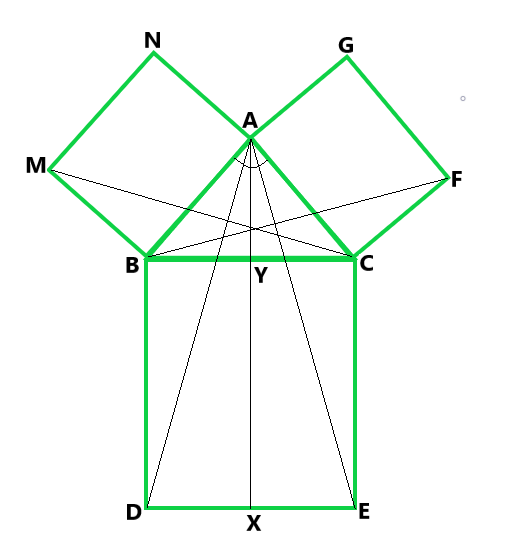Solution:

According to the question

ABC is a right-angled triangle at A, BCED, ACFG, and ABMN are squares on the sides BC, CA, and AB

(i) In ΔMBC and ΔABD,

MB = AB,

BC = BD,

∠MBC = ∠ABD

So, by SAS congruence, we have

ΔMBC ≅ ΔABD

Hence, ar(ΔMBC) = ar(ΔABD)

(ii) From the figure, triangle ABC and rectangle BYXD are on the same base BD

and between the same parallels AX and BD.

So, ar(ΔABD) = (1/2) ar(rect BYXD)

ar(rect BYXD) = 2ar(ΔABD)

From part (i)

Hence, ar(rect BYXD) = 2ar(ΔMBC)   ……(i)

(iii) From the figure, triangles MBC and square MBAN are on the same base MB and

between the same parallels MB and NC.

So, 2ar(ΔMBC) = ar(MBAN)  ……(ii)

From eq(i) and (ii), we get

Hence, ar(sq. MBAN) = ar(rect BYXD)

(iv) In ΔFCB and ACE,

FC = AC

CB = CE

∠FCB = ∠ACE

So, by SAS congruence,

Hence, ΔFCB ≅ ΔACE

(v) As we proved above

ΔFCB ≅ ΔACE

So, ar(ΔFCB) = ar(ΔACE)

From the figure, triangle ACE and rectangle CYXE are on the same base CE

and between same parallels CE and AX.

So, 2ar(ΔACE) = ar(CYXE)

Hence, 2ar(ΔFCB) = ar(ΔCYXE)        …..(iii)

(vi) From the figure, triangle FCb and rectangle FCAG are on the same base FC

and between the same parallels FC and BG.

So, 2ar(ΔFCB) = ar(FCAG)            ……(iv)

From eq(iii) and (iv), we get

ar(CYXE) = ar(ACFG)

(vii) In ΔACB, we have

Using Pythagoras theorem

BC2 = AB2 + AC2

BC × BD = AB × MB + AC × FC

Hence, ar(BCED) = ar(ABMN) + ar(ACFG)

Whether you're preparing for your first job interview or aiming to upskill in this ever-evolving tech landscape, GeeksforGeeks Courses are your key to success. We provide top-quality content at affordable prices, all geared towards accelerating your growth in a time-bound manner. Join the millions we've already empowered, and we're here to do the same for you. Don't miss out - check it out now!

Previous
Next